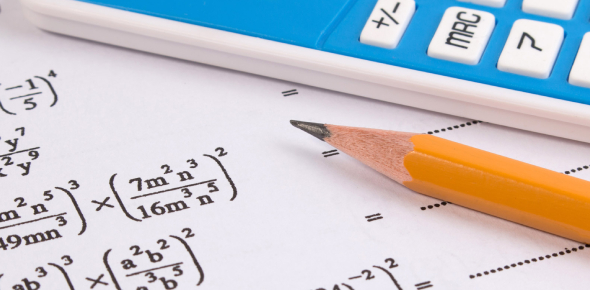# A Quick Mathematics Form I Test

12 Questions | Total Attempts: 14077SettingsWelcome, you all, here we have got you a quick mathematics quiz consisting of 12 questions. If you were looking for a Form 1 Mathematics test, then you must take it right now.

• 1.
Find the angle labeled X in the diagram:
• A.

50

• B.

82

• C.

98

• D.

102

• 2.
Find the value of y in the diagram in question 1.
• A.

50

• B.

82

• C.

98

• D.

48

• 3.
Amelia buys a crate of 30 eggs for \$20. If she sells each egg for 75 cents, state whether she made a profit or a loss and calculate the percentage (profit or loss).
• 4.
A man buys a car for \$40,000.00. Every year the value of the car depreciates by 10%. What is the car worth after 3 years?
• 5.
The Venn diagram below shows sets A and B, where A represents the set of items in Andy's schoolbag and B represents the set of items in Melissa's schoolbag. Find A n B (A intersect B)
• 6.
In the Venn diagram in question 8, which of the following represents A U B?
• A.

{color pencils, black ink pen, toy car, crayons, markers, red ink pen, coloring book}

• B.

{color pencils, black ink pen, toy car, crayons, markers, red ink pen, coloring book, pencil, blue ink pen, reading book, math book, copy book}

• C.

{color pencils, black ink pen, toy car, pencil, blue ink pen, reading book, math book, copy book}

• D.

{pencil, blue ink pen, reading book, math book, copy book, crayons, markers, red ink pen, coloring book}

• 7.
35/700 expressed as a percentage is:
• A.

50%

• B.

0.5%

• C.

5 %

• D.

0.05%

• 8.
The value of the 8 in the number 125, 085, 026 is:
• A.

8000

• B.

80000

• C.

800

• D.

800000

• 9.
13501 + 2793 equals
• A.

15293

• B.

15294

• C.

16293

• D.

16294

• 10.
50347 - 19805 equals
• A.

30542

• B.

40542

• C.

31542

• D.

41542

• 11.
2 x (5 - 1) + 3 - 1 / 5 =
• A.

2 3/5

• B.

2

• C.

10 4/5

• D.

9

• 12.
1.35 x 2.1 =
• A.

2.385

• B.

3.35

• C.

2.35

• D.

2.835

Related TopicsBack to top